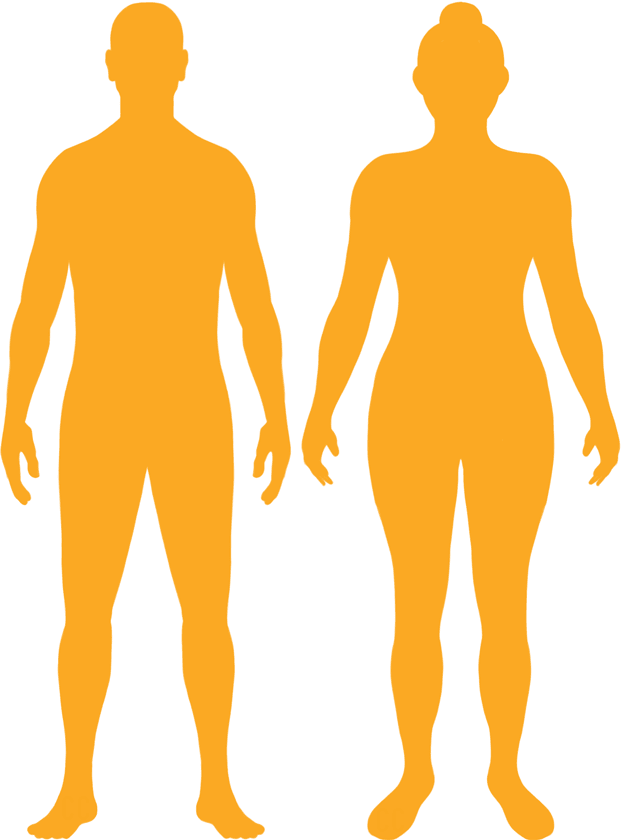# BMI Calculator

Result for 7'7" and 313 lbs.
Change Here.26.57

### Overweight BMI

At 7'7" and 313 Pounds your BMI is 26.57

### BMI Scale

 Under Normal Over Obese
-19 lbs
to reach a
normal BMI classification.
##### Normal BMI Ends:
294.5 lbs
+40 lbs
until you reach an obese BMI classification.
353.4 lbs

### BMI Grading Table2 for Height 7'7"

 Weight Weight Class Below 217.9 lbs Underweight 217.9 lbs - 294.5 lbs Normal Weight 294.5 lbs - 353.4 lbs Overweight 353.4 lbs and Above Obese

### Weight Loss

If you were seeking a normal BMI weight of 294.5lbs, and you lost two pounds a week4, then you could reach a normal BMI in
9.5 Weeks

### Overweight BMI Silhouette### Ideal Weight for a 7 foot 7 Male or Female2

The ideal weight range using the BMI for a male or female with a height of 7'7" is

### Between

217.9 lbs

and
294.5 lbs
How to Calculate BMI3 for 7'7" and 313 lbs
(7 x 12) + 7 = 91
(313 / (91 x 91)) x 703
= 26.571549329791
7 = foot | 7 = inch | 313 = weight lbs

### Conversion

• 7 foot 7 = 91 inches
• 7 foot 7 = 231.14cm
• 313 pounds = 22 stone 5 pounds
• 313 pounds = 141.97 kg
• 313 pounds = 141974.41 grams

### 7'7" and 313 lbs Summary

What is the BMI for a 7'7" and 313 lbs female? 26.57 BMI, Overweight.
What is the BMI for a 7'7" and 313 lbs male? 26.57 BMI, Overweight.
What is the ideal weight for a 7'7" female? Between: 217.9lbs and 294.5lbs
What is the ideal weight for a 7'7" male? Between: 217.9lbs and 294.5lbs
If I am 7ft 7in and weigh 313 lbs, is that a good weight for my height? Under the BMI classification, 313 lbs is classed as being Overweight.